22. Feb 2013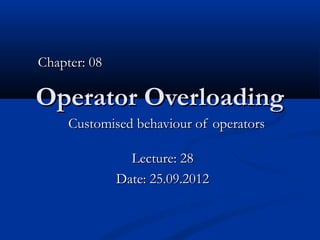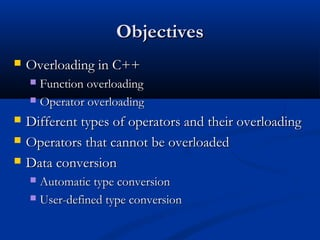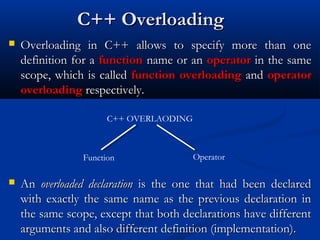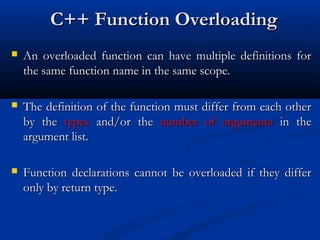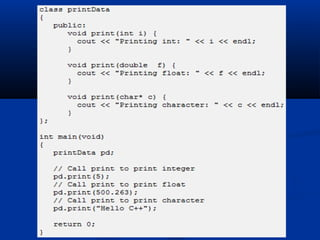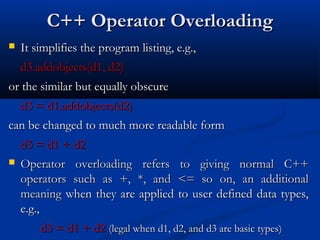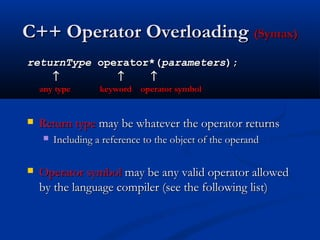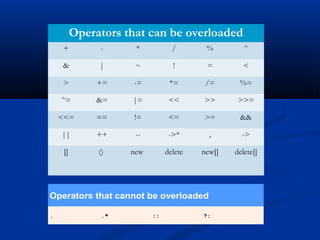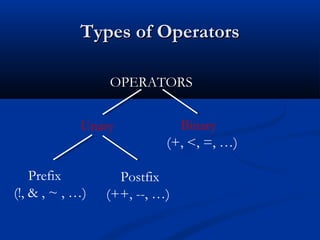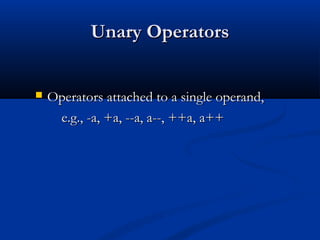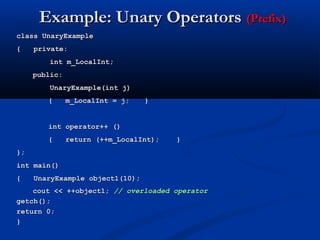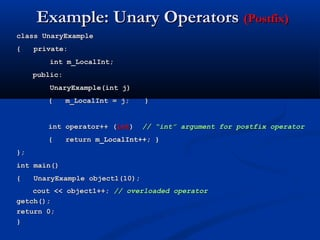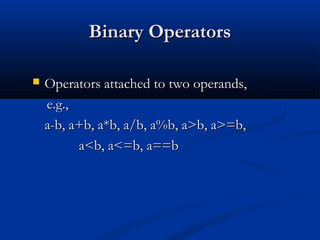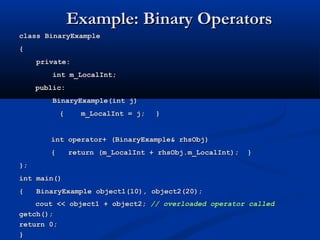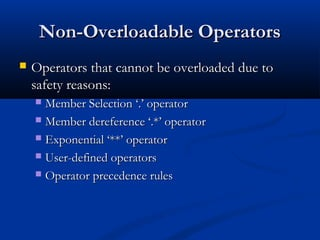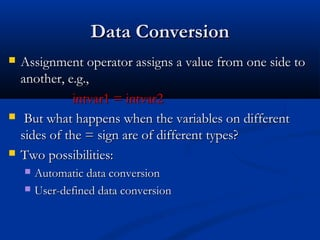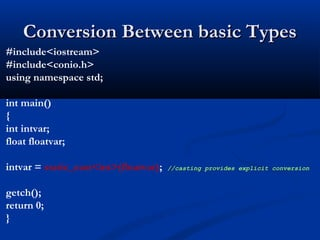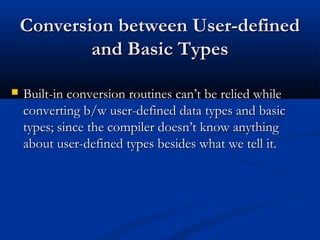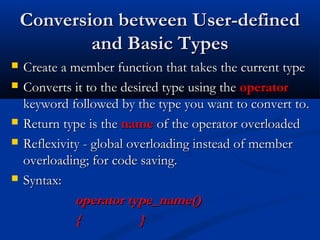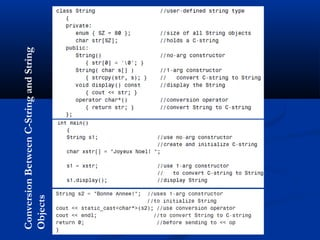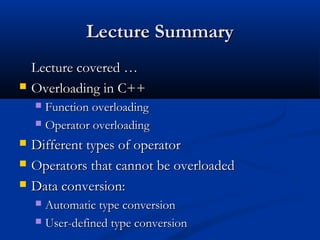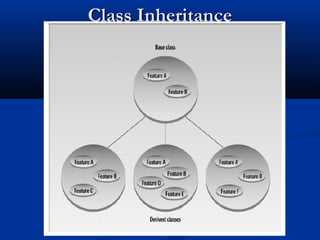1 von 23

• 1. Chapter: 08 Operator Overloading Customised behaviour of operators Lecture: 28 Date: 25.09.2012
• 3. C++ Overloading  Overloading in C++ allows to specify more than one definition for a function name or an operator in the same scope, which is called function overloading and operator overloading respectively. C++ OVERLAODING Function Operator  An overloaded declaration is the one that had been declared with exactly the same name as the previous declaration in the same scope, except that both declarations have different arguments and also different definition (implementation).
• 4. C++ Function Overloading  An overloaded function can have multiple definitions for the same function name in the same scope.  The definition of the function must differ from each other by the types and/or the number of arguments in the argument list.  Function declarations cannot be overloaded if they differ only by return type.
• 6. C++ Operator Overloading  It simplifies the program listing, e.g., d3.addobjects(d1, d2) or the similar but equally obscure d3 = d1.addobjects(d2) can be changed to much more readable form d3 = d1 + d2  Operator overloading refers to giving normal C++ operators such as +, *, and <= so on, an additional meaning when they are applied to user defined data types, e.g., d3 = d1 + d2 (legal when d1, d2, and d3 are basic types)
• 7. C++ Operator Overloading (Syntax) returnType operator*(parameters); ↑ ↑ ↑ any type keyword operator symbol  Return type may be whatever the operator returns  Including a reference to the object of the operand  Operator symbol may be any valid operator allowed by the language compiler (see the following list)
• 8. Operators that can be overloaded + - * / % ^ & | ~ ! = < > += -= *= /= %= ^= &= |= << >> >>= <<= == != <= >= && || ++ -- ->* , -> [] () new delete new[] delete[] Operators that cannot be overloaded . .* :: ?:
• 9. Types of Operators OPERATORS Unary Binary (+, <, =, …) Prefix Postfix (!, & , ~ , …) (++, --, …)
• 10. Unary Operators  Operators attached to a single operand, e.g., -a, +a, --a, a--, ++a, a++
• 11. Example: Unary Operators (Prefix) class UnaryExample { private: int m_LocalInt; public: UnaryExample(int j) { m_LocalInt = j; } int operator++ () { return (++m_LocalInt); } }; int main() { UnaryExample object1(10); cout << ++object1; // overloaded operator getch(); return 0; }
• 12. Example: Unary Operators (Postfix) class UnaryExample { private: int m_LocalInt; public: UnaryExample(int j) { m_LocalInt = j; } int operator++ (int) // “int” argument for postfix operator { return m_LocalInt++; } }; int main() { UnaryExample object1(10); cout << object1++; // overloaded operator getch(); return 0; }
• 13. Binary Operators  Operators attached to two operands, e.g., a-b, a+b, a*b, a/b, a%b, a>b, a>=b, a<b, a<=b, a==b
• 14. Example: Binary Operators class BinaryExample { private: int m_LocalInt; public: BinaryExample(int j) { m_LocalInt = j; } int operator+ (BinaryExample& rhsObj) { return (m_LocalInt + rhsObj.m_LocalInt); } }; int main() { BinaryExample object1(10), object2(20); cout << object1 + object2; // overloaded operator called getch(); return 0; }
• 15. Non-Overloadable Operators  Operators that cannot be overloaded due to safety reasons:  Member Selection ‘.’ operator  Member dereference ‘.*’ operator  Exponential ‘**’ operator  User-defined operators  Operator precedence rules
• 16. Data Conversion  Assignment operator assigns a value from one side to another, e.g., intvar1 = intvar2  But what happens when the variables on different sides of the = sign are of different types?  Two possibilities:  Automatic data conversion  User-defined data conversion
• 17. Conversion Between basic Types #include<iostream> #include<conio.h> using namespace std; int main() { int intvar; float floatvar; intvar = static_cast<int>(floatvar); //casting provides explicit conversion getch(); return 0; }
• 18. Conversion between User-defined and Basic Types  Built-in conversion routines can’t be relied while converting b/w user-defined data types and basic types; since the compiler doesn’t know anything about user-defined types besides what we tell it.
• 19. Conversion between User-defined and Basic Types  Create a member function that takes the current type  Converts it to the desired type using the operator keyword followed by the type you want to convert to.  Return type is the name of the operator overloaded  Reflexivity - global overloading instead of member overloading; for code saving.  Syntax: operator type_name() { }
• 20. Conversion Between C-String and String Objects
• 21. Lecture Summary Lecture covered …  Overloading in C++  Function overloading  Operator overloading  Different types of operator  Operators that cannot be overloaded  Data conversion:  Automatic type conversion  User-defined type conversion
• 22. Lecture Summary Lectures, books and so on will be updated at: http://www.itquest.tk/ (http://www.itquest.ucoz.com/) http://www.downloadbooks.mytestproject.co.cc/

### Hinweis der Redaktion

1. Student Book
2. Student Book
3. Student Book
4. Student Book
5. Student Book
6. Student Book Operator overloading is used for customised functionality of a an operator in a class. This a powerful tool in C++, where hundreds of lines of code can be slashed, if operator overloaded it done properly and efficiently.
7. Student Book Operator overloading is used for customised functionality of a an operator in a class. This a powerful tool in C++, where hundreds of lines of code can be slashed, if operator overloaded it done properly and efficiently.
8. Student Book Operator overloading is used for customised functionality of a an operator in a class. This a powerful tool in C++, where hundreds of lines of code can be slashed, if operator overloaded it done properly and efficiently.
9. Student Book Operator overloading is used for customised functionality of a an operator in a class. This a powerful tool in C++, where hundreds of lines of code can be slashed, if operator overloaded it done properly and efficiently.
10. Student Book The pre and post increment and decrement operators and overloading in different ways. This is because they have a different effect on objects and their values. e.g. a=14; cout &lt;&lt; a++; // will print 14 and increment a cout &lt;&lt; ++a; // will increment a and print 15
11. Student Book
12. Student Book
13. Student Book
14. Student Book
15. Student Book Exponential operator is reserved User-defined operators because of precedence problem
16. Student Book
17. Student Book
18. Student Book
19. Student Book
20. Student Book
21. Student Book
22. Student Book
23. Student Book